### Quadrilaterals Class 9th Mathematics AP Board Solution

##### Question 1.ABC is a triangle. D is a point on AB such that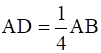and E is a point on AC such that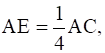If DE = 2 cm find BC.Answer: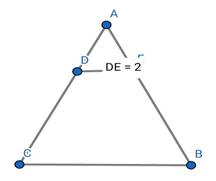Given:- DE=2cmFormula used:- Line joining midpoints of 2 sides of triangle will behalf of its 3rd side.Solution:-Assume mid points of line AB and AC is M and NThe formation of new triangle will be there Δ AMNAs;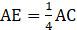⇒ AC=4AEand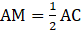∴ AM=2AEHence;E is the midpoint of AMSimilarlyD is the midpoint of AN→ In Δ AMNIf E,D are midpointsED=MNMN=2EDMN=2×2cm=4cm→ In Δ ABCIf M,N are midpointsAB=MNAB=2MNAB=2×4cm=8cmConclusion:-BC=8cmQuestion 2.ABCD is quadrilateral E, F, G and H are the midpoints of AB, BC, CD and DA respectively. Prove that EFGH is a parallelogram.Answer: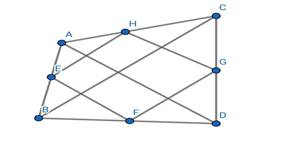Given:- ABCD is quadrilateral E, F, G and H are the midpoints ofAB, BC, CD and DA respectivelyFormula used:- Line joining midpoints of 2 sides of triangleIs parallel to 3rd sideSolution:-BD is diagonal of quadrilateralEH is the line joined by midpoints of triangle ABD,∴EH is parallel to BDGF is line joined by midpoints of side BC&BD of triangle BCD∴GF is parallel to BD⇒ If HE is parallel to BD and BD is parallel to GF∴ It gives HE is parallel to GF⇒ AC is another diagonal of quadrilateralGH is the line joined by midpoints of triangle ADC,∴GH is parallel to ACFE is line joined by midpoints of side BC&AB of triangle ABC∴FE is parallel to AC⇒ If GH is parallel to AC and AC is parallel to FE∴ It gives GH is parallel to FEAs HE||GF and GH||FE∴ EFGH is a parallelogramConclusion:-EFGH is a parallelogramQuestion 3.Show that the figure formed by joining the midpoints of sides of a rhombus successively is a rectangle.Answer: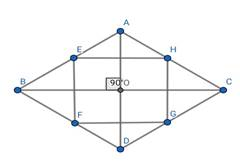Given:- ABCD is a rhombusE,F,G,H are the mid points of AB,BC,CD,DAFormula used:- Line joining midpoints of 2 sides of triangleIs parallel and half of 3rd sideSolution:-BD is diagonal of rhombusEH is the line joined by midpoints of triangle ABD,∴EH is parallel and half of BDGF is line joined by midpoints of side BC&BD of triangle BCD∴GF is parallel and half BD⇒ If HE is parallel to BD and BD is parallel to GF∴ It gives HE is parallel to GF⇒ If HE is half of BD and GF is also half of BD∴ It gives HE is equal to GF⇒ AC is another diagonal of rhombusGH is the line joined by midpoints of triangle ADC,∴GH is parallel to AC∴GH is half of ACFE is line joined by midpoints of side BC&AB of triangle ABC∴FE is parallel to AC∴ FE is half of AC⇒ If GH is parallel to AC and AC is parallel to FE∴ It gives GH is parallel to FE⇒ If GH is half of AC and FE is also half of AC∴ It gives GH is equal to FEAs diagonals of rhombus intersect at 90°And AC is parallel to FE and GHAll angles of EFGH is 90°All angles are 90° and opposite sides are equal and parallelConclusion:-EFGH is a rectangleQuestion 4.In a parallelogram ABCD, E and F are the midpoints of the sides AB and DC respectively. Show that the line segments AF and EC trisect the diagonal BD.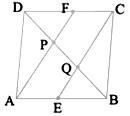Answer:Given:- ABCD is a parallelogramE and F are the midpoints of the sides AB and DC respectivelyFormula used:- Line drawn through midpoint of one side of triangleParallel to other side , bisect the 3rd side.Solution:-As ABCD is parallelogramAB=CD and AB||CD;⇒ AE=CF and AE||CF [E and F are the midpoints of AB and CD]In quadrilateral AECF⇒ AE=CF and AE||CFAECF is a parallelogram.∵ Quadrilateral having one pair of side equal and parallel are parallelogramIf AECF is a parallelogram∴ AF||CEHence ;PF||CQ and AP||QE [As AF=AP+PF and CE=CQ+QE ]In Δ DQCDF=FC [F is midpoint]PF||CQThen;P is midpoint of DQDP=PQ∵ Line drawn through midpoint of one side of triangle Parallel toother side , bisect the 3rd sideIn Δ APBAE=EB [E is midpoint]AP||QEThen;Q is midpoint of PBPQ=QB∵ Line drawn through midpoint of one side of triangle Parallel toother side , bisect the 3rd sideIf DP=PQ and PQ=QBThen DP=PQ=QBConclusion:-Line segments AF and EC trisect the diagonal BD.Question 5.Show that the line segments joining the midpoints of the opposite sides of a quadrilateral and bisect each other.Answer: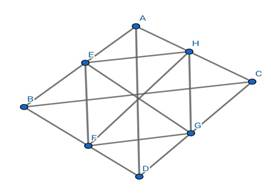Given:- ABCD is a quadrilateralFormula used:- Line joining midpoints of 2 sides of triangleIs parallel and half of 3rd sideSolution:-BD is diagonal of quadrilateralEH is the line joined by midpoints of triangle ABD,∴EH is parallel and half of BDGF is line joined by midpoints of side BC&BD of triangle BCD∴GF is parallel and half BD⇒ If HE is parallel to BD and BD is parallel to GF∴ It gives HE is parallel to GF⇒ If HE is half of BD and GF is also half of BD∴ It gives HE is equal to GF⇒ AC is another diagonal of quadrilateralGH is the line joined by midpoints of triangle ADC,∴GH is parallel to AC∴GH is half of ACFE is line joined by midpoints of side BC&AB of triangle ABC∴FE is parallel to AC∴ FE is half of AC⇒ If GH is parallel to AC and AC is parallel to FE∴ It gives GH is parallel to FE⇒ If GH is half of AC and FE is also half of AC∴ It gives GH is equal to FEIf both opposite sides are parallel and equalThen, the quadrilateral is parallelogramIf EFGH is parallelogramThen their diagonal bisect each otherIf diagonal of parallelogram is the line joining midpoint of opposite sides of quadrilateralThen;Line joining midpoints of opposite sides of quadrilateral bisect each other.Conclusion:-Lines joining midpoints of opposite sides of quadrilateral bisect each otherQuestion 6.ABC is a triangle right angled at C. A line through the midpoint M of hypotenuse AB and Parallel to BC intersects AC at D. Show that(i) D is the midpoint of AC(ii) MD ⊥ AC(iii) CM = MA =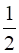AB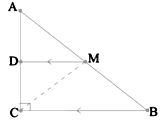Answer:Given:- ACB is right angle triangleM is midpoint of ABDM||CBFormula used:- Line drawn through midpoint of one side of triangleParallel to other side , bisect the 3rd side* SAS congruency propertyIf 2 sides and angle between the two sides of boththe triangle are equal, then both triangle are congruentSolution:-(1) In Δ ABCM is midpoint of ABAnd DM||CB∴ D is midpoint of ACAD=DC∵ Line drawn through midpoint of one side of triangleParallel to other side , bisect the 3rd side(2) In Δ ABCDM||CB∠ ADM=∠ ACB [Corresponding angles]∠ ACB =90°∠ ADM=90°(3) In Δ ADM and Δ DMC⇒ DM=DM [Common in both triangles ]⇒ AD=DC [D is the midpoint]As ADC is straight line∠ ADM+∠ MDC=180°∠ MDC=180° - ∠ ADM∠ MDC=90°⇒ ∠ ADM=∠ MDCHence;In Δ ADM ≅ Δ DMC∴ CM=MA⇒ MA=AB [M is midpoint of AB]∴ CM=MA=AB

PDF FILE TO YOUR EMAIL IMMEDIATELY PURCHASE NOTES & PAPER SOLUTION. @ Rs. 50/- each (GST extra)

HINDI ENTIRE PAPER SOLUTION

MARATHI PAPER SOLUTION

SSC MATHS I PAPER SOLUTION

SSC MATHS II PAPER SOLUTION

SSC SCIENCE I PAPER SOLUTION

SSC SCIENCE II PAPER SOLUTION

SSC ENGLISH PAPER SOLUTION

SSC & HSC ENGLISH WRITING SKILL

HSC ACCOUNTS NOTES

HSC OCM NOTES

HSC ECONOMICS NOTES

HSC SECRETARIAL PRACTICE NOTES

# 2019 Board Paper Solution

HSC ENGLISH SET A 2019 21st February, 2019

HSC ENGLISH SET B 2019 21st February, 2019

HSC ENGLISH SET C 2019 21st February, 2019

HSC ENGLISH SET D 2019 21st February, 2019

SECRETARIAL PRACTICE (S.P) 2019 25th February, 2019

HSC XII PHYSICS 2019 25th February, 2019

CHEMISTRY XII HSC SOLUTION 27th, February, 2019

OCM PAPER SOLUTION 2019 27th, February, 2019

HSC MATHS PAPER SOLUTION COMMERCE, 2nd March, 2019

HSC MATHS PAPER SOLUTION SCIENCE 2nd, March, 2019

SSC ENGLISH STD 10 5TH MARCH, 2019.

HSC XII ACCOUNTS 2019 6th March, 2019

HSC XII BIOLOGY 2019 6TH March, 2019

HSC XII ECONOMICS 9Th March 2019

SSC Maths I March 2019 Solution 10th Standard11th, March, 2019

SSC MATHS II MARCH 2019 SOLUTION 10TH STD.13th March, 2019

SSC SCIENCE I MARCH 2019 SOLUTION 10TH STD. 15th March, 2019.

SSC SCIENCE II MARCH 2019 SOLUTION 10TH STD. 18th March, 2019.

SSC SOCIAL SCIENCE I MARCH 2019 SOLUTION20th March, 2019

SSC SOCIAL SCIENCE II MARCH 2019 SOLUTION, 22nd March, 2019

XII CBSE - BOARD - MARCH - 2019 ENGLISH - QP + SOLUTIONS, 2nd March, 2019

# HSCMaharashtraBoardPapers2020

(Std 12th English Medium)

HSC ECONOMICS MARCH 2020

HSC OCM MARCH 2020

HSC ACCOUNTS MARCH 2020

HSC S.P. MARCH 2020

HSC ENGLISH MARCH 2020

HSC HINDI MARCH 2020

HSC MARATHI MARCH 2020

HSC MATHS MARCH 2020

# SSCMaharashtraBoardPapers2020

(Std 10th English Medium)

English MARCH 2020

HindI MARCH 2020

Hindi (Composite) MARCH 2020

Marathi MARCH 2020

Mathematics (Paper 1) MARCH 2020

Mathematics (Paper 2) MARCH 2020

Sanskrit MARCH 2020

Sanskrit (Composite) MARCH 2020

Science (Paper 1) MARCH 2020

Science (Paper 2)

Geography Model Set 1 2020-2021

MUST REMEMBER THINGS on the day of Exam

Are you prepared? for English Grammar in Board Exam.

Paper Presentation In Board Exam

How to Score Good Marks in SSC Board Exams

Tips To Score More Than 90% Marks In 12th Board Exam

How to write English exams?

How to prepare for board exam when less time is left

How to memorise what you learn for board exam

No. 1 Simple Hack, you can try out, in preparing for Board Exam

How to Study for CBSE Class 10 Board Exams Subject Wise Tips?

JEE Main 2020 Registration Process – Exam Pattern & Important Dates

NEET UG 2020 Registration Process Exam Pattern & Important Dates

How can One Prepare for two Competitive Exams at the same time?

8 Proven Tips to Handle Anxiety before Exams!